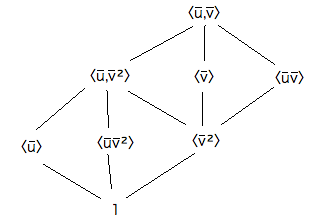If you find any mistakes, please make a comment! Thank you.

## Compute the subgroup lattice of a quotient of modular group of order 16

Solution to Abstract Algebra by Dummit & Foote 3rd edition Chapter 3.3 Exercise 3.3.6

Let $M = \langle u,v \rangle$ be the modular group of order 16 described in Exercise 3.1.19. Prove that $\langle v^4 \rangle$ is normal in $M$ and use the lattice isomorphism theorem to draw the lattice of subgroups of $M/\langle v^4 \rangle$. Which group of order 8 has the same subgroup lattice? Use generators and relations to determine the isomorphism type of $M/\langle v^4 \rangle$.

Solution: Note that $vv^4v^{-1} = v^4 \in \langle v^4 \rangle$ and $$uv^4u^{-1} = uv^4u = u^2v^{20} = v^4 \in \langle v^4 \rangle;$$ by Exercise 3.1.19, $\langle v^4 \rangle$ is normal in $M$.

By the Lattice isomorphism theorem, the subgroup lattice of $M/\langle v^4 \rangle$ is as follows.Subgroup lattice of a quotient of the modular group of order 16

The group $\mathbb{Z}/(2) \times \mathbb{Z}/(4)$ has the same subgroup lattice, and in fact $$M/\langle v^4 \rangle = \langle \overline{u}, \overline{v} \ |\ \overline{u}^2 = \overline{v}^4 = 1, \overline{v}\overline{u} = \overline{u} \overline{v} \rangle,$$ so that $M/\langle v^4 \rangle \cong \mathbb{Z}/(2) \times \mathbb{Z}/(4)$.